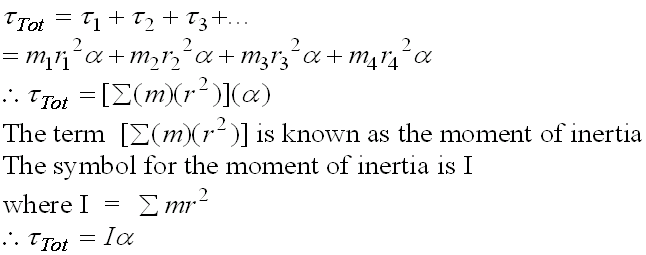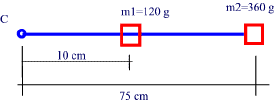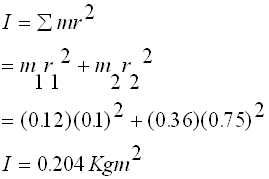### Moments of Inertia

• Inertia is defineed as the property of an object to resist changes on motion.
• The ability of an object to continue to stay at rest or  in its existing state of rest or uniform motion in a straight line.
• Unless that state is changed by an external, unbalanced force.
• Rotational Inertia measures the ability of an object to resist rotating about a fixed pint around a circular path.
• The moment of inertia is the ability of an object to resist a change in its angular acceleration.
• A torque is a force applied to an object, in a circular path, capable of alterning its moment of inertia or angular acceleration.

The total rotational torque taken at the center of gravity of a complex rotating object can be expressed as the sum of all torques acting on individual parts of the rotating object.
The illustration below is of a rectangular object rotating about a point C.
The object can be taken a s a whole or broken up into smaller masses, each rotating about  slightly different radius from the center of rotation.For the system above, for example,Example:

Two masses are suspended on a nylon thread of negligible mass as shown.
Find the moment of Inertia and the total torque of the system if it completes 20 revolutions in 10 s.m1 = 0.12 Kg
m2 = 0.36 Kg
r1 = 0.1 m
r2 = 0.75 m

Solution:

a) Find the moment of inertia Ib) Find the total Torque

First find the angular acceleration a